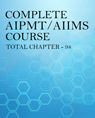Click to Chat

1800-1023-196

+91-120-4616500

CART 0

• 0

MY CART (5)

Use Coupon: CART20 and get 20% off on all online Study Material

ITEM
DETAILS
MRP
DISCOUNT
FINAL PRICE
Total Price: Rs.

There are no items in this cart.
Continue Shopping
        There are 2 formulas for escape vel,in what condition should we use the first formula and where should we use the second
one year agoSaurabh Koranglekar
4320 Points
							Dear studenthttps://www.askiitians.com/forums/Mechanics/10/28695/gravity.htmplease find the link

one year ago
							HELLO THERE! There are two formulas, that’s correct! But both are inter-related. Here I’m showing you how to derive the second formula from the first one. We know, that Gravitational Potention Energy (U) = where, M = mass of Earth, m = mass of the satellite (or escaping object), and R = Radius of Earth. And, escape energy (E) =  Let the escape velocity of the satellite be v, then it’s Kinetic energy =  Now, this Kinetic energy = Escape energy when the satellite is escaping. So, ….....(i) This is the first formula. Now, from the relation between G (Universal Gravitational Constant) and g (acceleration due to gravity), we know that: Let’s modify the first formula that we have obtained (Equation 1):We have, So, these are the two formulas, and you need to use them according to the data given in the question. If only the radius of earth (or radius of the planet from which the object is escaping is given, then you can use the second formula). If the mass of the earth or the planet from which the object is leaving is given, the first formula can be used. G has a constant value. HOPE MY ANSWER IS SATISFACTORY...Thanks!

one year ago
Think You Can Provide A Better Answer ?

## Other Related Questions on AIPMT

View all Questions »### Course Features

• 731 Video Lectures
• Revision Notes
• Previous Year Papers
• Mind Map
• Study Planner
• NCERT Solutions
• Discussion Forum
• Test paper with Video Solution### Course Features

• 728 Video Lectures
• Revision Notes
• Test paper with Video Solution
• Mind Map
• Study Planner
• NCERT Solutions
• Discussion Forum
• Previous Year Exam Questions